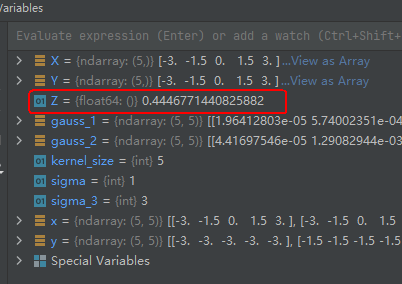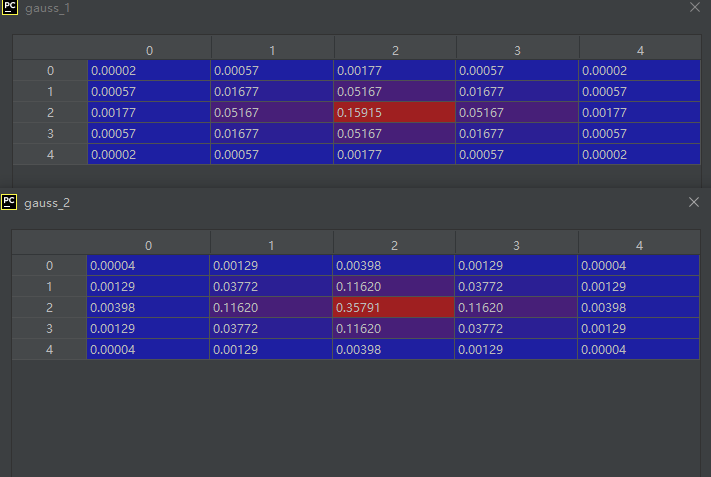https://blog.csdn.net/u013066730/article/details/123112159

1 为什么高斯滤波能够让图像实现模糊化？

2 为什么很多文章中说生成高斯核时，我们通常取的范围就是-3个标准差到+3个标准差的范围。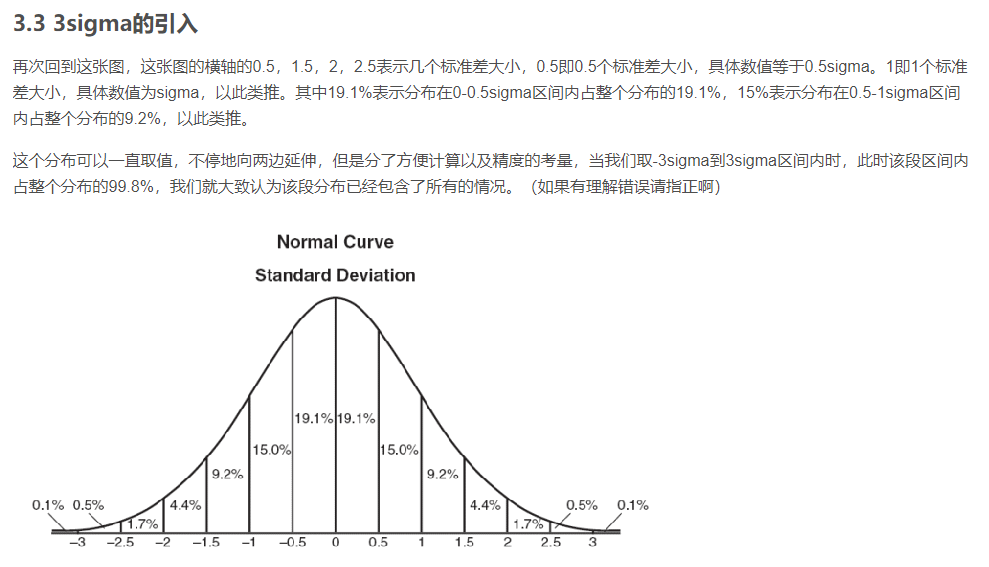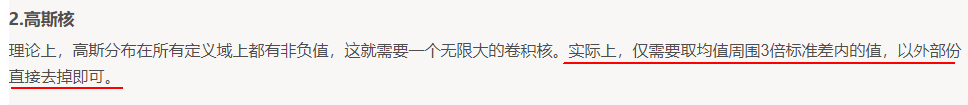3 下面两幅截图中的159、273是怎么来的？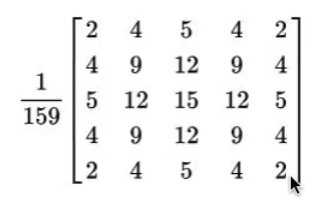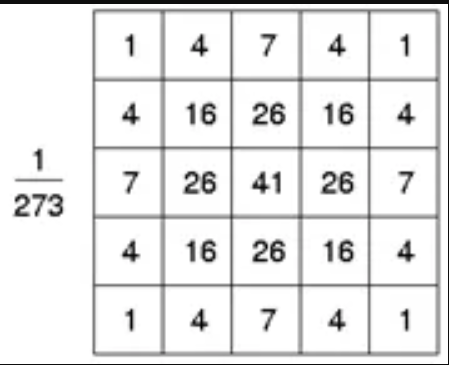``````>> 2+4+5+4+2+4+9+12+9+4+5+12+15+12+5+4+9+12+9+4+2+4+5+4+2

ans =

159

>>
``````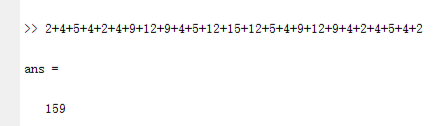``````>> 1+4+7+4+1+4+16+26+16+4+7+26+41+26+7+4+16+26+16+4+1+4+7+4+1

ans =

273

>>
``````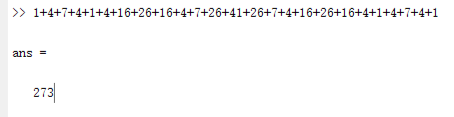PS:什么是相关运算、什么又是卷积运算？答：看下面这篇博文就能搞清楚了。
https://blog.csdn.net/zhuwei0710/article/details/68169317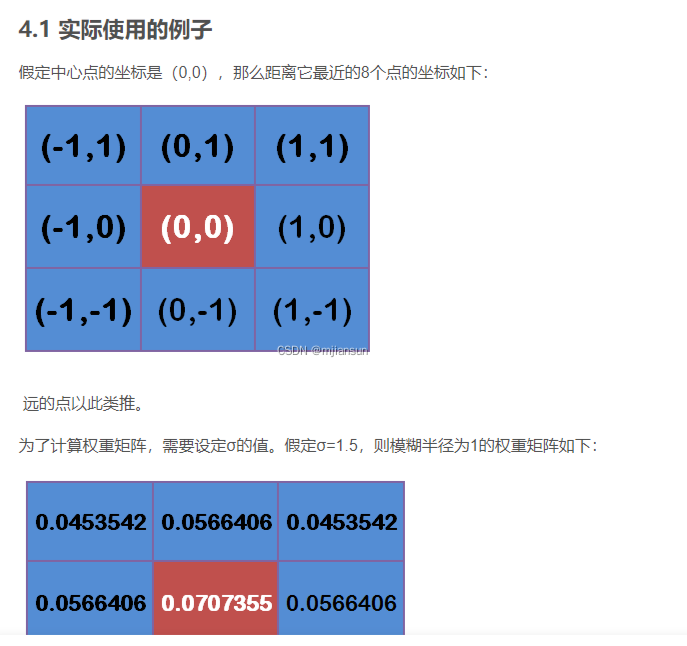4 是否需要仔细分析博文https://blog.csdn.net/u013066730/article/details/123112159对高斯核分离的原理介绍?

5 标准差、均值相同，那么3阶高斯核、5阶高斯是否是惟一的？

6 下面是自己用Python写的生成高斯核的代码：

``````#!/usr/bin/env python
# -*- coding: utf-8 -*-
#作者微信/QQ 2487872782
#有问题可以联系作者交流
# 代码中涉及到函数meshgrid()的使用,不清楚地可以参考我的博文
# https://blog.csdn.net/wenhao_ir/article/details/124124717

import numpy as np
import sys

kernel_size = 5
sigma = 1

if sigma == 0:
print('Error!The value of sigma cannot be zero.')
sys.exit()
else:
sigma_3 = 3 * sigma
X = np.linspace(-sigma_3, sigma_3, kernel_size)
Y = np.linspace(-sigma_3, sigma_3, kernel_size)
x, y = np.meshgrid(X, Y)
gauss_1 = 1 / (2 * np.pi * sigma ** 2) * np.exp(- (x ** 2 + y ** 2) / (2 * sigma ** 2))
Z = gauss_1.sum() #计算归一化系数
gauss_2 = (1/Z)*gauss_1

``````